Like   Tweet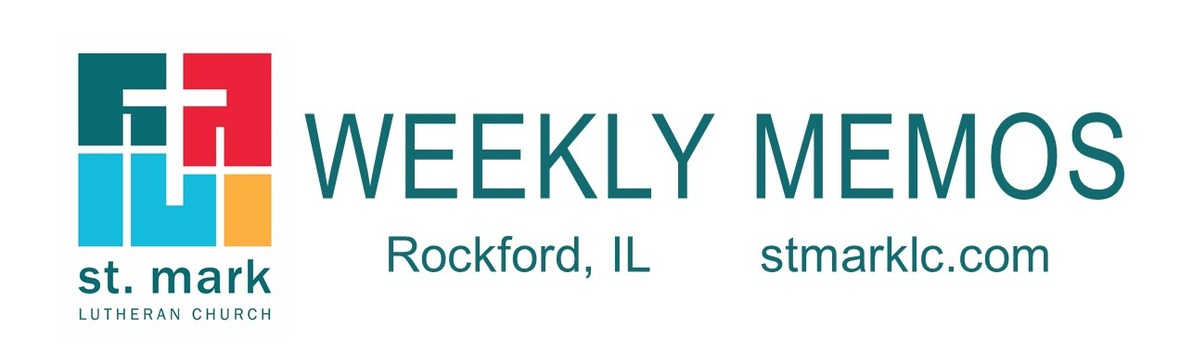table div table+table div table{width:100%;padding:0}table div table+table div table img{width:96.23%;padding:0;float:none}table div table+table div table td{width:100%;padding:0 1.88% 18px}/* styles */## Pastoral Reflection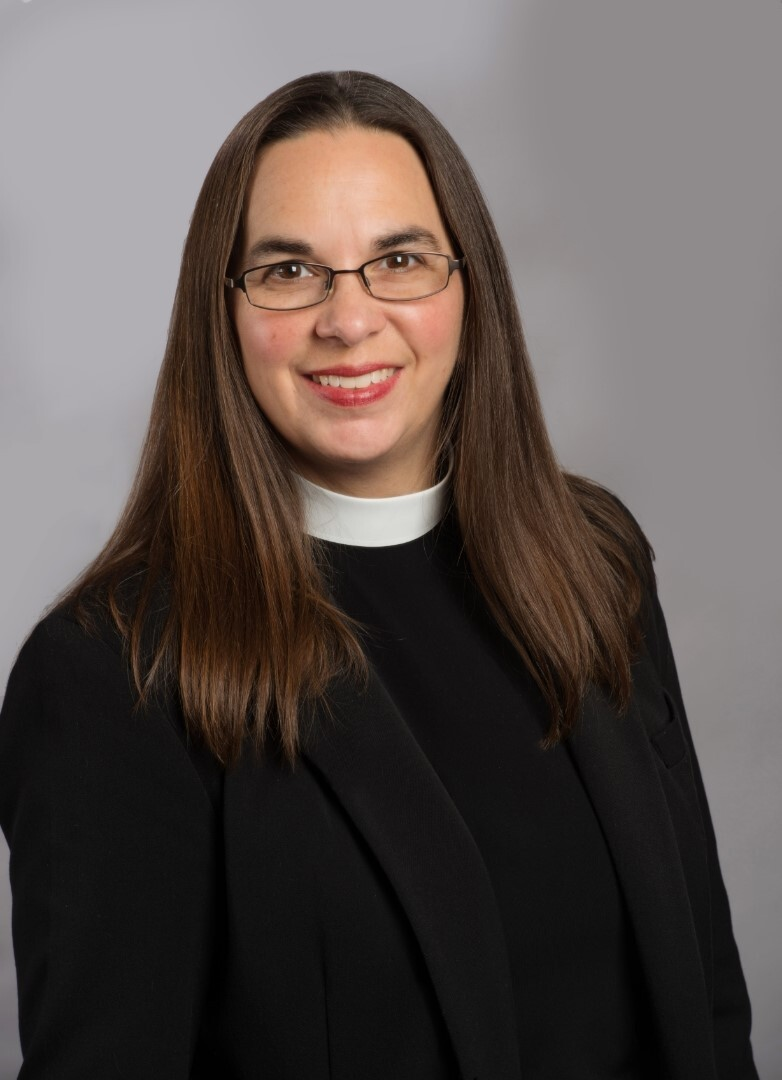Ordination Reflection
Pastor Katrina Steingraeber
A few days after my ordination I was asked how it feels to be an official pastor. My reply was, it feels a bit surreal, a bit overwhelming, and a bit like normal.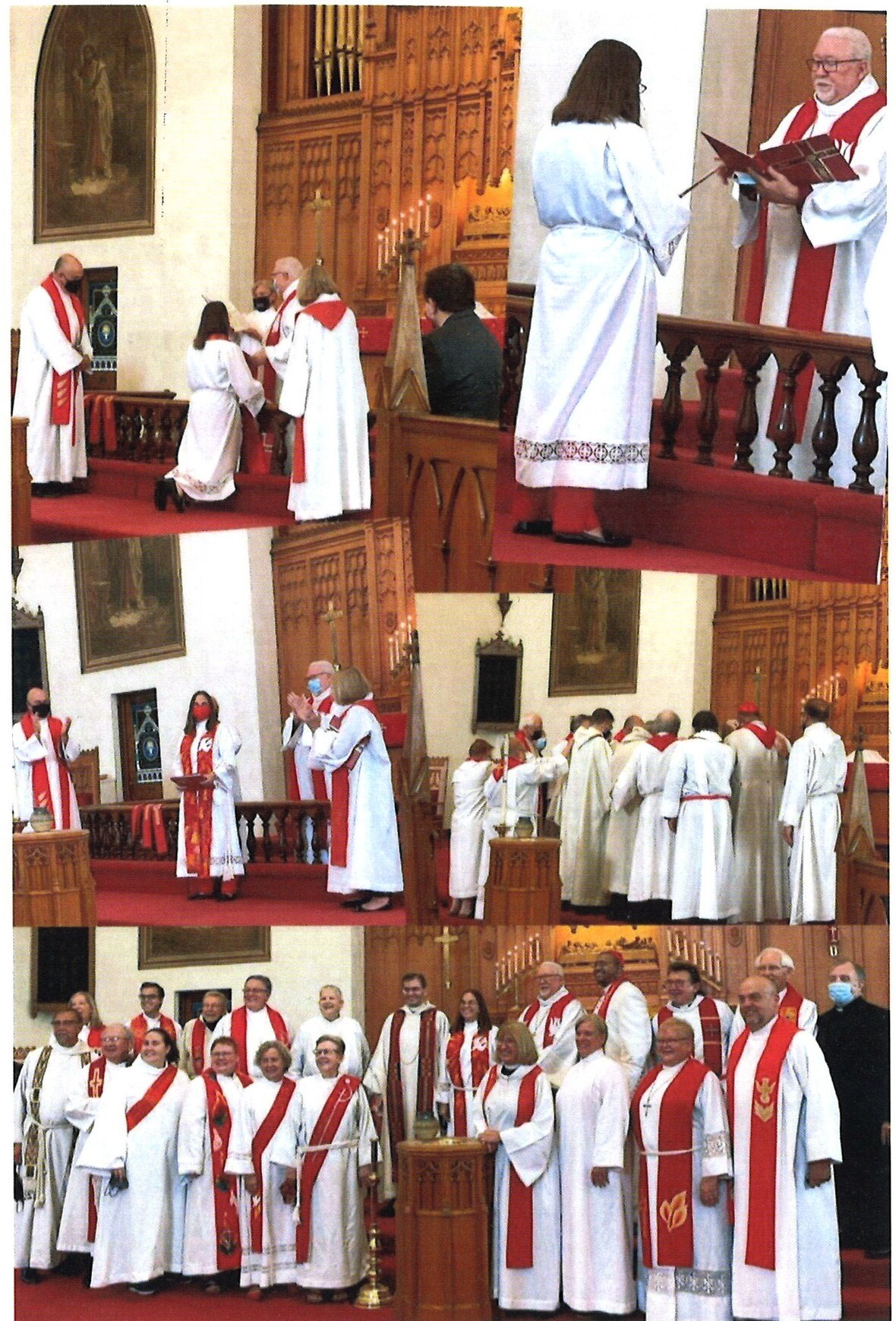## Sunday, September 12

9:30 A.M. Fellowship before service - Coffee & Donut Hour
10:30 A.M. Rally Sunday Worship Service outdoors under the tent
11:30 A.M. Catered Lunch

## Monday, September 13

5:00 P.M. Children's Ministry

## Tuesday, September 14

10:00 A.M. Staff Meeting
11:30 A.M. St. Mark Duplicate Bridge

## Wednesday, September 15

9:00 A.M. Women's Bible Study meets at Stockholm Inn
9:30 A.M. St. Mark Ringers
6:30 P.M. Confirmation
7:00 P.M. Voices of St. Mark

## Thursday, September 16

9:00 A.M. Cherry Valley Bridge Group
6:30 P.M. Emotions Anonymous
6:30 P.M. Blue Lights

## Friday, September 17

7:30 A.M. St. Mark Men's Breakfast at Denny's Restaurant
7:00 P.M. Grand Groove Hotel Concert under the tent outdoors

## Sunday, September 19 Backpack Blessing & Installation of Sunday School Teachers at 9:00

9:00 A.M. Indoor Worship Live-Stream in the Sanctuary
10:00 A.M. Fellowship Hour with coffee and donuts
10:10 A.M. Sunday School Begins
10:10 A.M. Learning Hour
11:00 A.M. Worship Outdoors

 table div table+table+table+table+table+table+table+table div table{width:100%;padding:0}table div table+table+table+table+table+table+table+table div table img{width:96.23%;padding:0;float:none}table div table+table+table+table+table+table+table+table div table td{width:100%;padding:0 1.88% 18px}/* styles */## News and Events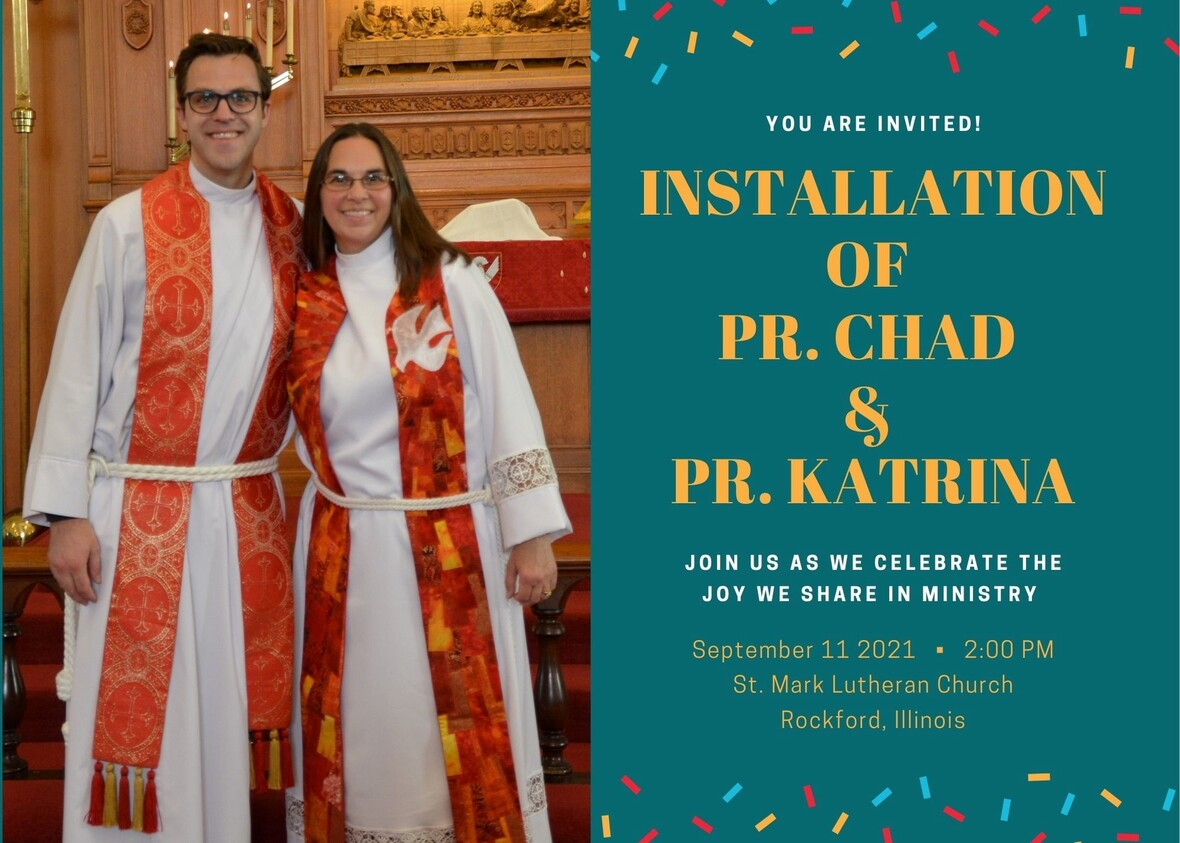table div table+table+table+table+table+table+table+table+table+table+table div table{width:100%;padding:0}table div table+table+table+table+table+table+table+table+table+table+table div table img{width:96.23%;padding:0;float:none}table div table+table+table+table+table+table+table+table+table+table+table div table td{width:100%;padding:0 1.88% 18px}/* styles *//* styles */ 60th Anniversary Festival Worship On Saturday, September 11 at 2:00 P.M. we will install both Pastor Chad and Pastor Katrina and celebrate our 60th anniversary as a congregation. St. Mark called Rev. Chad McKenna to be our senior pastor on August 30, 2020; we called Rev. Katrina Steingraeber to be our associate pastor on June 6, 2021. If you are unable to attend this service, we will have it live-streamed at this link. During this full Rally weekend, this is our only traditional service. Our Rally Sunday worship will occur on September 12th at 10:30 under the tent, and is not live-streamed. It will be followed by a free barbecue lunch, and we hope you will join us if you are able!
 table div table+table+table+table+table+table+table+table+table+table+table+table+table div table{width:100%;padding:0}table div table+table+table+table+table+table+table+table+table+table+table+table+table div table img{width:96.23%;padding:0;float:none}table div table+table+table+table+table+table+table+table+table+table+table+table+table div table td{width:100%;padding:0 1.88% 18px}/* styles *//* styles */ Rally Sunday Worship September 12 9:30 am - Fellowship Hour with coffee and donuts 10:30 am - Worship outdoors under the tent 11:30 am - Catered Lunch outdoors
 table div table+table+table+table+table+table+table+table+table+table+table+table+table+table+table div table{width:100%;padding:0}table div table+table+table+table+table+table+table+table+table+table+table+table+table+table+table div table img{width:96.23%;padding:0;float:none}table div table+table+table+table+table+table+table+table+table+table+table+table+table+table+table div table td{width:100%;padding:0 1.88% 18px}/* styles */table div table+table+table+table+table+table+table+table+table+table+table+table+table+table+table+table+table div table{width:100%;padding:0}table div table+table+table+table+table+table+table+table+table+table+table+table+table+table+table+table+table div table img{width:96.23%;padding:0;float:none}table div table+table+table+table+table+table+table+table+table+table+table+table+table+table+table+table+table div table td{width:100%;padding:0 1.88% 18px}/* styles *//* styles */ 60th Anniversary Banquet and Rally Weekend Below is the schedule for the weekend.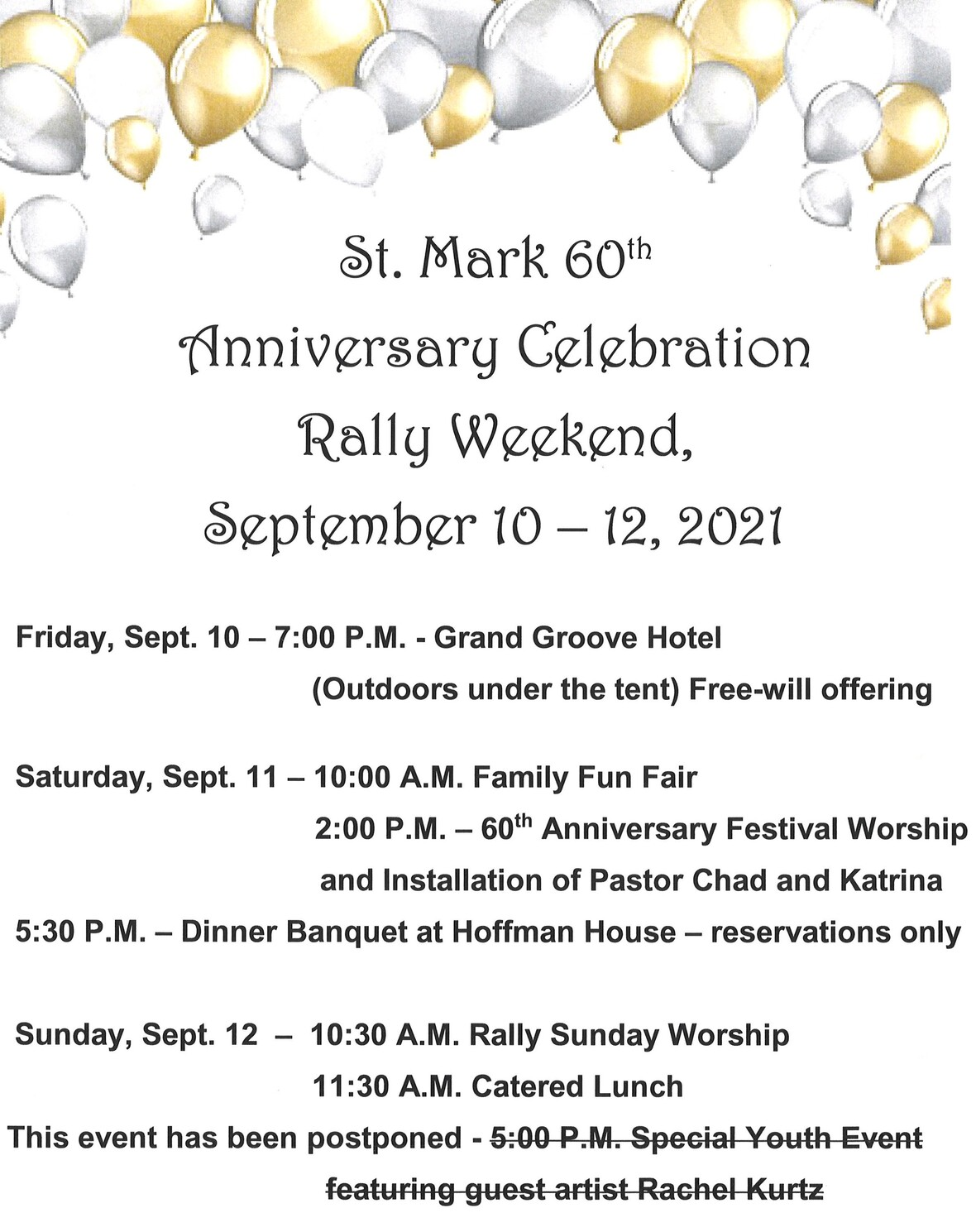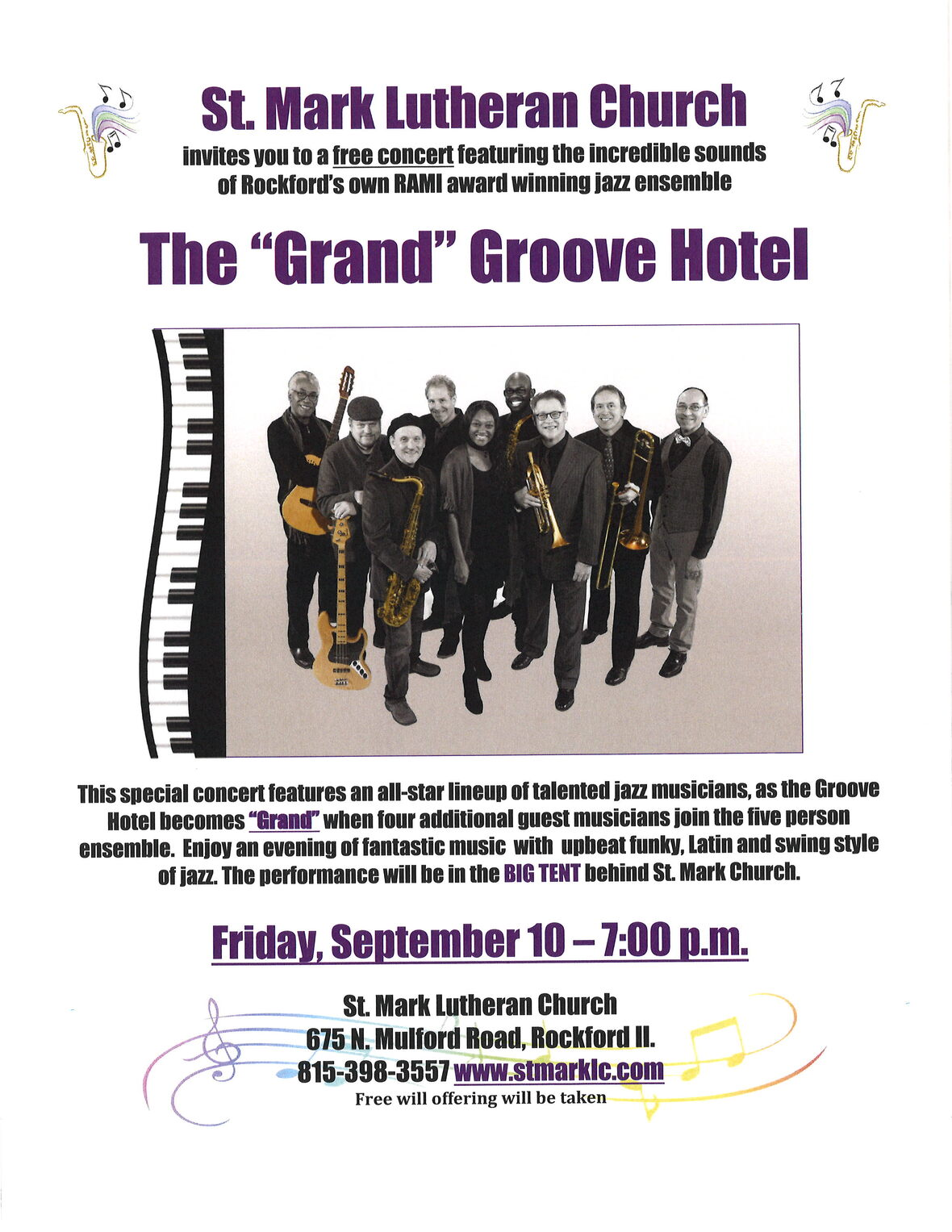table div table+table+table+table+table+table+table+table+table+table+table+table+table+table+table+table+table+table+table+table+table div table{width:100%;padding:0}table div table+table+table+table+table+table+table+table+table+table+table+table+table+table+table+table+table+table+table+table+table div table img{width:96.23%;padding:0;float:none}table div table+table+table+table+table+table+table+table+table+table+table+table+table+table+table+table+table+table+table+table+table div table td{width:100%;padding:0 1.88% 18px}/* styles */table div table+table+table+table+table+table+table+table+table+table+table+table+table+table+table+table+table+table+table+table+table+table+table div table{width:100%;padding:0}table div table+table+table+table+table+table+table+table+table+table+table+table+table+table+table+table+table+table+table+table+table+table+table div table img{width:96.23%;padding:0;float:none}table div table+table+table+table+table+table+table+table+table+table+table+table+table+table+table+table+table+table+table+table+table+table+table div table td{width:100%;padding:0 1.88% 18px}/* styles */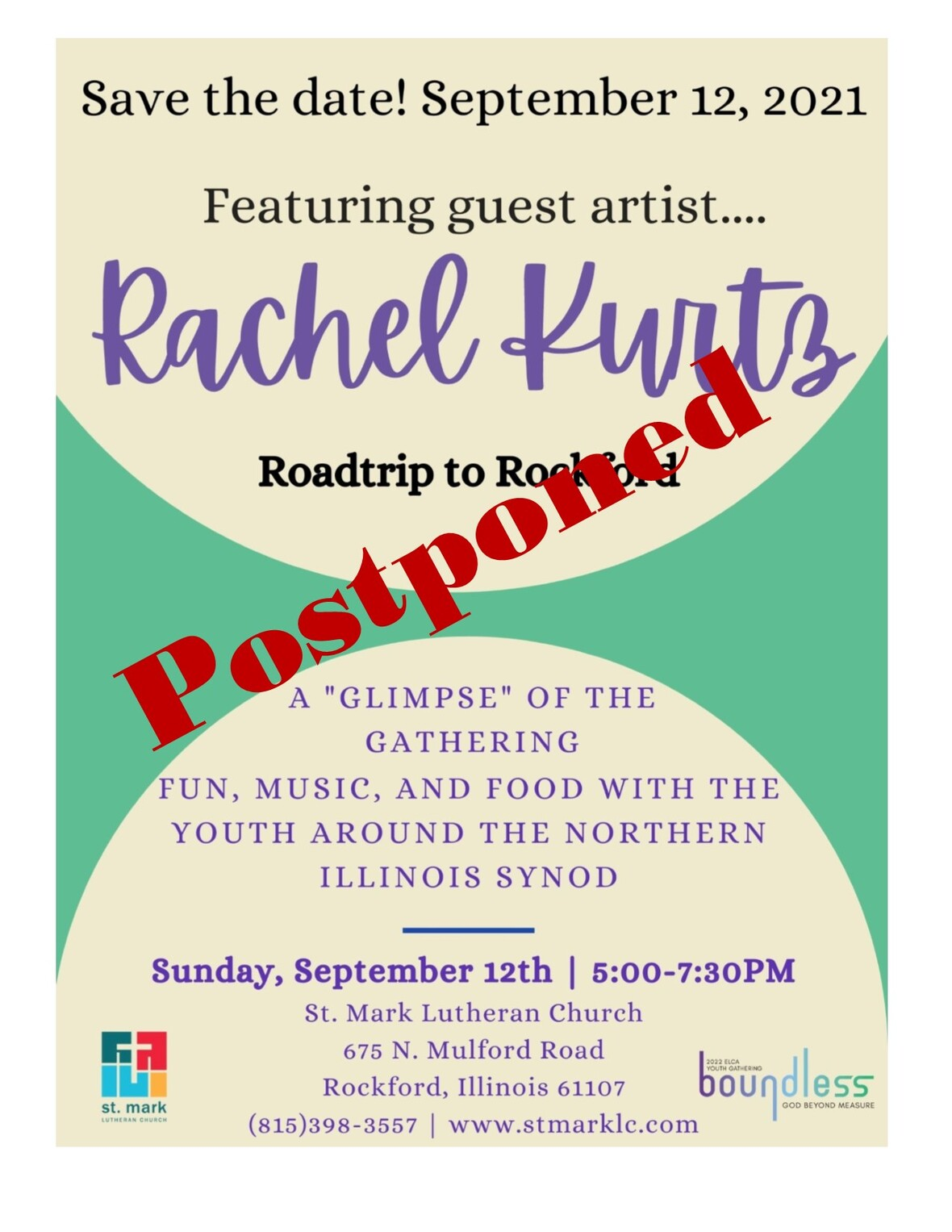table div table+table+table+table+table+table+table+table+table+table+table+table+table+table+table+table+table+table+table+table+table+table+table+table+table div table{width:100%;padding:0}table div table+table+table+table+table+table+table+table+table+table+table+table+table+table+table+table+table+table+table+table+table+table+table+table+table div table img{width:96.23%;padding:0;float:none}table div table+table+table+table+table+table+table+table+table+table+table+table+table+table+table+table+table+table+table+table+table+table+table+table+table div table td{width:100%;padding:0 1.88% 18px}/* styles */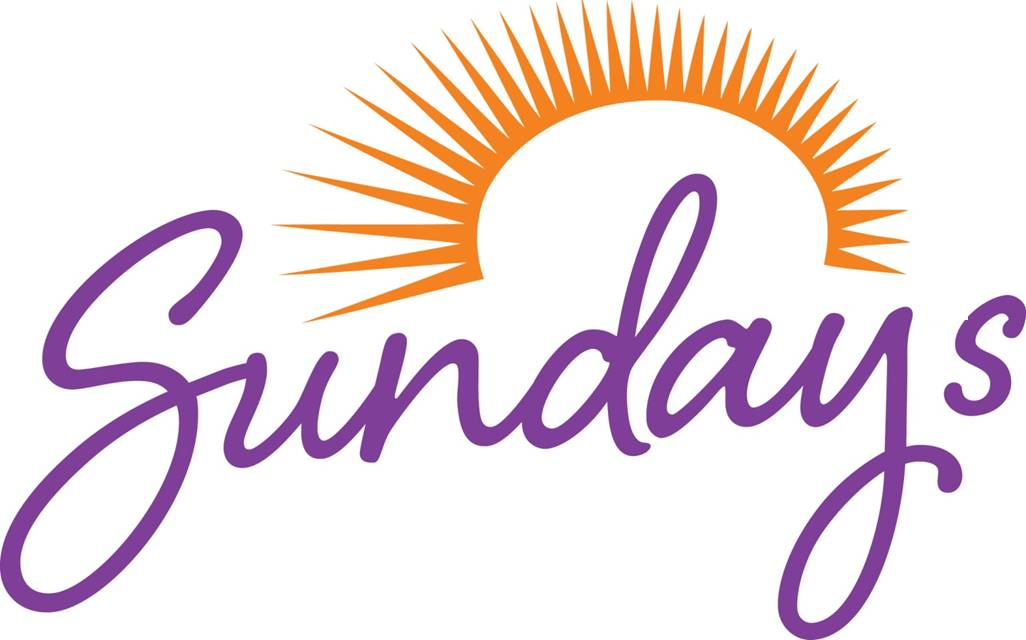Fall Sunday Schedule
Beginning on September 19th we will have Traditional Worship at 9:00 am, a Learning Hour at 10:10 am, and a Praise Service at 11:00 am. During that learning hour, we will have Sunday School downstairs, Fellowship over coffee and donuts in both the café and Fellowship Hall, and a brand new Adult Forum in the Adult Ministry Room. This fall, the content of the Adult Forum will change from week to week, and you can expect to see opportunities for Bible studies, book studies, presentations from local agencies, and group discussions. Read Pastor Chad's full Pastoral Reflection from last week here.

 table div table+table+table+table+table+table+table+table+table+table+table+table+table+table+table+table+table+table+table+table+table+table+table+table+table+table+table div table{width:100%;padding:0}table div table+table+table+table+table+table+table+table+table+table+table+table+table+table+table+table+table+table+table+table+table+table+table+table+table+table+table div table img{width:96.23%;padding:0;float:none}table div table+table+table+table+table+table+table+table+table+table+table+table+table+table+table+table+table+table+table+table+table+table+table+table+table+table+table div table td{width:100%;padding:0 1.88% 18px}/* styles *//* styles */ Our Sunday morning Adult Forum begins next week, September 19 during the learning hour at 10:10 am. The theme during September is “How Has Covid Changed You?” Each week will focus on a different aspect of this theme. Bring your coffee and a donut to the Adult Ministry room for this open discussion led by one of our pastors. Sunday School and Fellowship also occur during this time between services.
 table div table+table+table+table+table+table+table+table+table+table+table+table+table+table+table+table+table+table+table+table+table+table+table+table+table+table+table+table+table div table{width:100%;padding:0}table div table+table+table+table+table+table+table+table+table+table+table+table+table+table+table+table+table+table+table+table+table+table+table+table+table+table+table+table+table div table img{width:96.23%;padding:0;float:none}table div table+table+table+table+table+table+table+table+table+table+table+table+table+table+table+table+table+table+table+table+table+table+table+table+table+table+table+table+table div table td{width:100%;padding:0 1.88% 18px}/* styles */Family Photo
One of many great things about our Instant Church Directory is that you can send an updated family photo anytime. Some of you have had babies, kids are getting older, and some of our families have grown.

Click on this link to submit your photo. If you want to stop by the church office, we can take the picture for you.

 table div table+table+table+table+table+table+table+table+table+table+table+table+table+table+table+table+table+table+table+table+table+table+table+table+table+table+table+table+table+table+table div table{width:100%;padding:0}table div table+table+table+table+table+table+table+table+table+table+table+table+table+table+table+table+table+table+table+table+table+table+table+table+table+table+table+table+table+table+table div table img{width:96.23%;padding:0;float:none}table div table+table+table+table+table+table+table+table+table+table+table+table+table+table+table+table+table+table+table+table+table+table+table+table+table+table+table+table+table+table+table div table td{width:100%;padding:0 1.88% 18px}/* styles */Because We CARE!
Parents of students in college or in the military please send in their address. We would like to collect all addresses by October 1. Please e-mail melinda@stmarklc.com We also appreciate any donations towards shipping. Thank you!

 table div table+table+table+table+table+table+table+table+table+table+table+table+table+table+table+table+table+table+table+table+table+table+table+table+table+table+table+table+table+table+table+table+table div table{width:100%;padding:0}table div table+table+table+table+table+table+table+table+table+table+table+table+table+table+table+table+table+table+table+table+table+table+table+table+table+table+table+table+table+table+table+table+table div table img{width:96.23%;padding:0;float:none}table div table+table+table+table+table+table+table+table+table+table+table+table+table+table+table+table+table+table+table+table+table+table+table+table+table+table+table+table+table+table+table+table+table div table td{width:100%;padding:0 1.88% 18px}/* styles */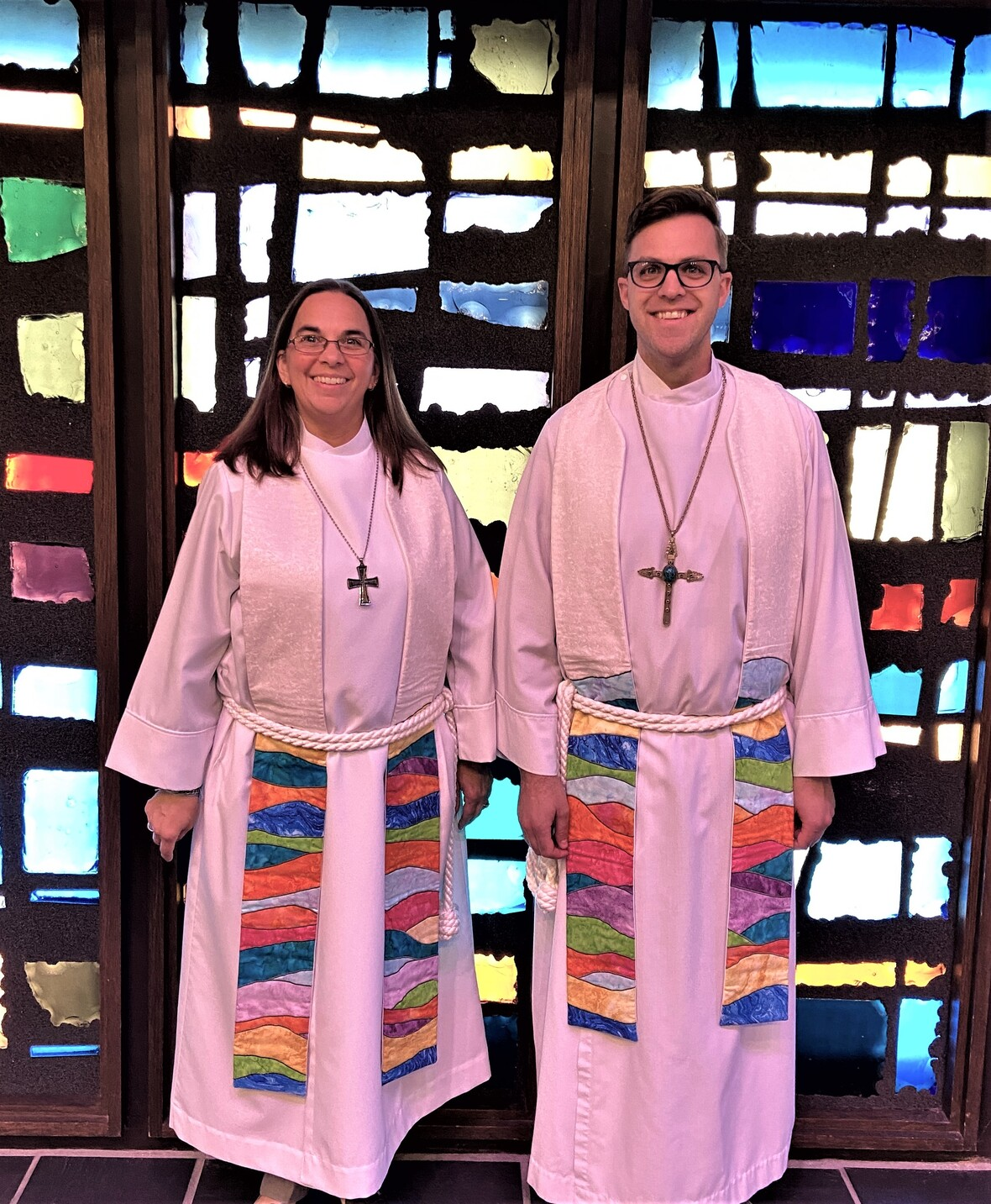Thank you St. Mark Women, for the beautiful stoles. That was a wonderful and special surprise last Sunday. Thank you for lifting up women in ministry and for acknowledging the hard work of this past year.

With gratitude,

 table div table+table+table+table+table+table+table+table+table+table+table+table+table+table+table+table+table+table+table+table+table+table+table+table+table+table+table+table+table+table+table+table+table+table+table div table{width:100%;padding:0}table div table+table+table+table+table+table+table+table+table+table+table+table+table+table+table+table+table+table+table+table+table+table+table+table+table+table+table+table+table+table+table+table+table+table+table div table img{width:96.23%;padding:0;float:none}table div table+table+table+table+table+table+table+table+table+table+table+table+table+table+table+table+table+table+table+table+table+table+table+table+table+table+table+table+table+table+table+table+table+table+table div table td{width:100%;padding:0 1.88% 18px}/* styles */By now a lot of us have the church directory app or an online account and love it!

If you haven't gotten around to it, take a few minutes to follow the instructions below. You don't need to have a smartphone to have the directory, you can create an account on your computer and use the directory online. It is simple and easy to use. We do have paper copies available for you if you don't have access to a computer, just call the office to let us know.

Instant Church Directory is a simple way to view your Church Directory. Never deal with an outdated directory again, any changes made by church staff are immediately synced to the app.

Mobile App Login Instructions for Android & Kindle Devices

We also have a form for you to complete to make sure we have the most current information for the directory. There are paper
forms at church or click on the link to Church Directory Form

If you need any assistance or have questions, please call Jill Davenport at 815-398-3557 or stop by the office.

 table div table+table+table+table+table+table+table+table+table+table+table+table+table+table+table+table+table+table+table+table+table+table+table+table+table+table+table+table+table+table+table+table+table+table+table+table+table div table{width:100%;padding:0}table div table+table+table+table+table+table+table+table+table+table+table+table+table+table+table+table+table+table+table+table+table+table+table+table+table+table+table+table+table+table+table+table+table+table+table+table+table div table img{width:96.23%;padding:0;float:none}table div table+table+table+table+table+table+table+table+table+table+table+table+table+table+table+table+table+table+table+table+table+table+table+table+table+table+table+table+table+table+table+table+table+table+table+table+table div table td{width:100%;padding:0 1.88% 18px}/* styles *//* styles */ Celebrating 60 Years of St. Mark September “collection” we are honoring our memories of St. Mark Preschool. We want to hear from you! We hope to collect sixty memories, stories, and pictures to post. Even if you didn’t have someone that attended the pre-school, I bet you know someone that did! St. Mark Preschool began operating on January 4, 1971 with 20 children and quickly grew to be the premier preschool in the Rockford area with over 200 children enrolled every year. Sadly the preschool closed in May 2013 after 42 years of providing exceptional early childhood education with a Christian atmosphere. Send in your favorite memory, a story, or a picture. You may email general@stmarklc.com or bring to the church office.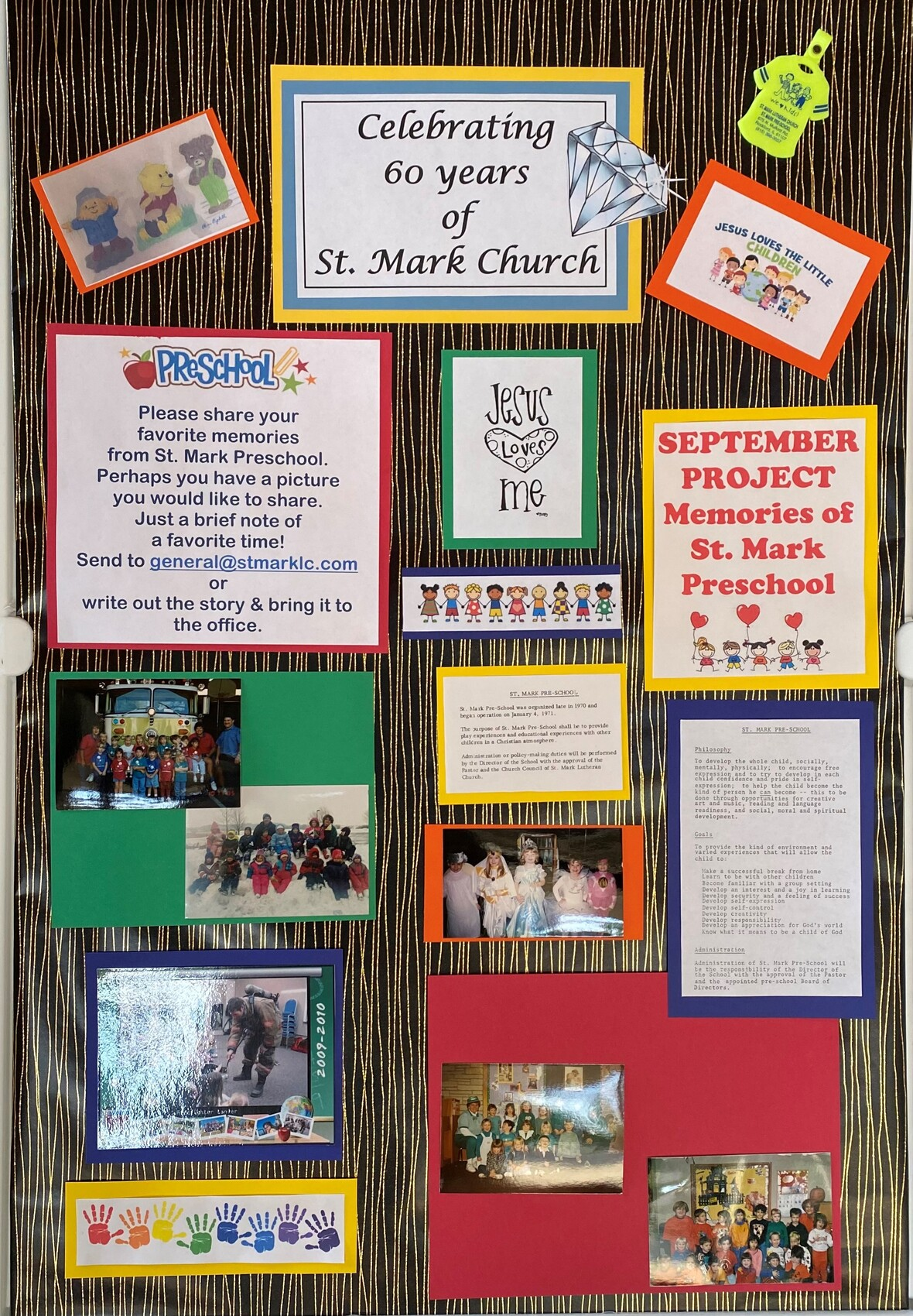table div table+table+table+table+table+table+table+table+table+table+table+table+table+table+table+table+table+table+table+table+table+table+table+table+table+table+table+table+table+table+table+table+table+table+table+table+table+table+table+table div table{width:100%;padding:0}table div table+table+table+table+table+table+table+table+table+table+table+table+table+table+table+table+table+table+table+table+table+table+table+table+table+table+table+table+table+table+table+table+table+table+table+table+table+table+table+table div table img{width:96.23%;padding:0;float:none}table div table+table+table+table+table+table+table+table+table+table+table+table+table+table+table+table+table+table+table+table+table+table+table+table+table+table+table+table+table+table+table+table+table+table+table+table+table+table+table+table div table td{width:100%;padding:0 1.88% 18px}/* styles *//* styles */ (60 Years of St. Mark) A memory: My son, Kevin, attended St Mark Preschool from 1992 to 1994. The preschool was very well regarded in our community and was an excellent faith based learning opportunity with a wonderful kindergarten readiness program. Mrs.Kalousek, Mrs. Iverson, Mrs. Swanson, and Mrs. Lundstrom loved their students and made learning fun. As an added bonus, a few parents of my son's classmates have remained my best friends for over 25 years. St Mark Preschool was a wonderful start to my son's education.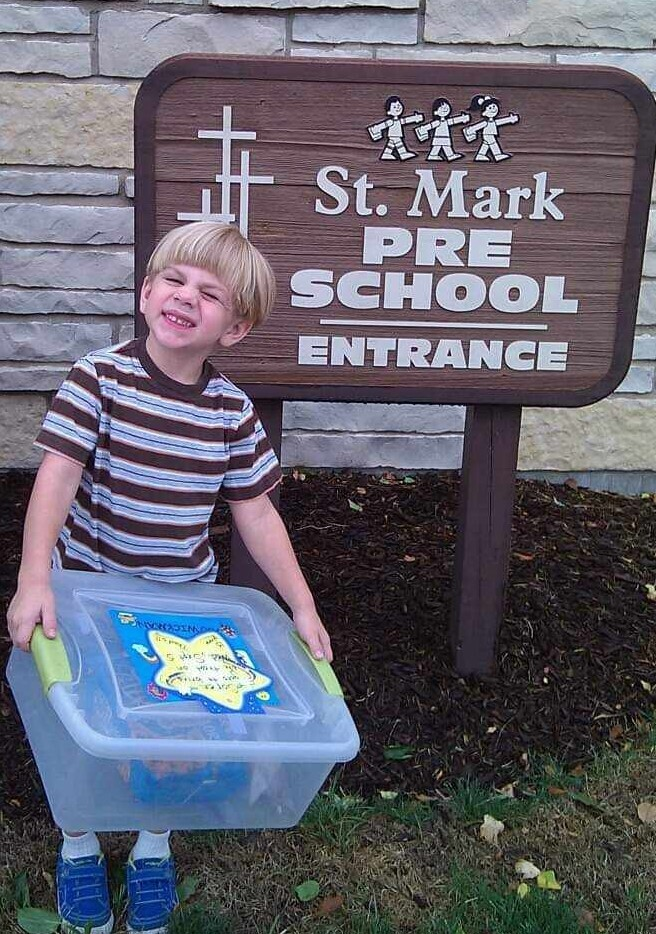Soren Steingraeber
Who remembers the "snack" box?

 table div table+table+table+table+table+table+table+table+table+table+table+table+table+table+table+table+table+table+table+table+table+table+table+table+table+table+table+table+table+table+table+table+table+table+table+table+table+table+table+table+table+table+table div table{width:100%;padding:0}table div table+table+table+table+table+table+table+table+table+table+table+table+table+table+table+table+table+table+table+table+table+table+table+table+table+table+table+table+table+table+table+table+table+table+table+table+table+table+table+table+table+table+table div table img{width:96.23%;padding:0;float:none}table div table+table+table+table+table+table+table+table+table+table+table+table+table+table+table+table+table+table+table+table+table+table+table+table+table+table+table+table+table+table+table+table+table+table+table+table+table+table+table+table+table+table+table div table td{width:100%;padding:0 1.88% 18px}/* styles */Confirmation News
Confirmation is just around the corner. Classes will begin on September 15, 2021 at 6:30 pm. Please fill out the registration form at this link.
I look forward to meeting you all and exploring the Bible during Confirmation Class. Peace, Pastor Katrina

 table div table+table+table+table+table+table+table+table+table+table+table+table+table+table+table+table+table+table+table+table+table+table+table+table+table+table+table+table+table+table+table+table+table+table+table+table+table+table+table+table+table+table+table+table+table div table{width:100%;padding:0}table div table+table+table+table+table+table+table+table+table+table+table+table+table+table+table+table+table+table+table+table+table+table+table+table+table+table+table+table+table+table+table+table+table+table+table+table+table+table+table+table+table+table+table+table+table div table img{width:96.23%;padding:0;float:none}table div table+table+table+table+table+table+table+table+table+table+table+table+table+table+table+table+table+table+table+table+table+table+table+table+table+table+table+table+table+table+table+table+table+table+table+table+table+table+table+table+table+table+table+table+table div table td{width:100%;padding:0 1.88% 18px}/* styles */Share God’s Love With Our Kids!
If you enjoy spending time with kids and learning more about God by examining the Bible through activities and conversation, then you should be a Sunday school teacher! No previous teaching experience is required – you will have all of the information and supplies that you need before you ever step into the classroom. Lessons are well written and easy to use, and Julie is always available to answer questions or give any other support you may need. And, team teaching means you and your teammates set a weekly or monthly rotation that works for your schedules.

Being a Sunday school teacher is a fun and joyful way to engage with kids, gain a new understanding of the Bible readings we all hear each week, and be a part of the St. Mark community in a deep and meaningful way.

 table div table+table+table+table+table+table+table+table+table+table+table+table+table+table+table+table+table+table+table+table+table+table+table+table+table+table+table+table+table+table+table+table+table+table+table+table+table+table+table+table+table+table+table+table+table+table+table div table{width:100%;padding:0}table div table+table+table+table+table+table+table+table+table+table+table+table+table+table+table+table+table+table+table+table+table+table+table+table+table+table+table+table+table+table+table+table+table+table+table+table+table+table+table+table+table+table+table+table+table+table+table div table img{width:96.23%;padding:0;float:none}table div table+table+table+table+table+table+table+table+table+table+table+table+table+table+table+table+table+table+table+table+table+table+table+table+table+table+table+table+table+table+table+table+table+table+table+table+table+table+table+table+table+table+table+table+table+table+table div table td{width:100%;padding:0 1.88% 18px}/* styles */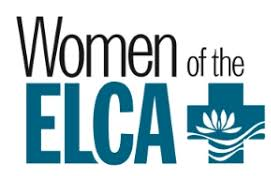Lydia Circle
Lydia Circle will meet on September 27, 2021, at 7:00 pm at church in the kitchen. Remember how the church kitchen was beautifully remodeled and finished just before Covid hit? Well, it has never really been put back together and organized since then. So, we will meet on the 27th to do a short bible study and then we will tackle the kitchen, label-maker and all. And if reorganizing a kitchen sounds like a nightmare to you, that's okay! Come anyway for the bible study and just stay to chat. No RSVP needed. Hope to see you there.

 table div table+table+table+table+table+table+table+table+table+table+table+table+table+table+table+table+table+table+table+table+table+table+table+table+table+table+table+table+table+table+table+table+table+table+table+table+table+table+table+table+table+table+table+table+table+table+table+table+table div table{width:100%;padding:0}table div table+table+table+table+table+table+table+table+table+table+table+table+table+table+table+table+table+table+table+table+table+table+table+table+table+table+table+table+table+table+table+table+table+table+table+table+table+table+table+table+table+table+table+table+table+table+table+table+table div table img{width:96.23%;padding:0;float:none}table div table+table+table+table+table+table+table+table+table+table+table+table+table+table+table+table+table+table+table+table+table+table+table+table+table+table+table+table+table+table+table+table+table+table+table+table+table+table+table+table+table+table+table+table+table+table+table+table+table div table td{width:100%;padding:0 1.88% 18px}/* styles */The Wednesday morning Women's Bible Study will resume for the 2021-2022 term on September 15th. We will begin the new year with a breakfast get-together at the Stockholm Inn at 9:00 am. This is open to everyone- those who have been attending and those who are interested in joining us! This will be a good way to get ready for the Fall term. The regular meeting of the Bible Study will be on September 22nd at 9:00 in the Conference Room. We will begin with a study of Galatians. Paul's letter to the Galatians is one argument after another about the amazing grace we receive from God. The Bible Study is open to all the women of St. Mark and friends. The Bible Study is based on Scripture, discussion, and life experiences from the participants. If you would like more information call Deb Iverson.The Wednesday morning Men's Bible Study will resume on September 22 at 9:00 a.m. in the cafe. We will brainstorm Bible study ideas on the 22nd.

 table div table+table+table+table+table+table+table+table+table+table+table+table+table+table+table+table+table+table+table+table+table+table+table+table+table+table+table+table+table+table+table+table+table+table+table+table+table+table+table+table+table+table+table+table+table+table+table+table+table+table+table+table div table{width:100%;padding:0}table div table+table+table+table+table+table+table+table+table+table+table+table+table+table+table+table+table+table+table+table+table+table+table+table+table+table+table+table+table+table+table+table+table+table+table+table+table+table+table+table+table+table+table+table+table+table+table+table+table+table+table+table div table img{width:96.23%;padding:0;float:none}table div table+table+table+table+table+table+table+table+table+table+table+table+table+table+table+table+table+table+table+table+table+table+table+table+table+table+table+table+table+table+table+table+table+table+table+table+table+table+table+table+table+table+table+table+table+table+table+table+table+table+table+table div table td{width:100%;padding:0 1.88% 18px}/* styles */Do you like to sew, but don’t have time to commit to meetings?
I am looking for a few individuals who like to sew to help make port pillows for some of our area cancer centers. The pillows are simple to make and all the supplies that you would need to sew a pillow are included in Ziploc baggies with instructions, and you only need a sewing machine and thread (any color). Sewing one pillow only takes a few minutes. You only need to sew the pillow pieces together, someone else will stuff and complete the pillows. Each baggie has supplies for 5 pillows. If you are interested, there is a basket with kits and a finished port pillow in fellowship hall. Questions: please contact Nancy Leonard 815-978-1406.

 table div table+table+table+table+table+table+table+table+table+table+table+table+table+table+table+table+table+table+table+table+table+table+table+table+table+table+table+table+table+table+table+table+table+table+table+table+table+table+table+table+table+table+table+table+table+table+table+table+table+table+table+table+table+table div table{width:100%;padding:0}table div table+table+table+table+table+table+table+table+table+table+table+table+table+table+table+table+table+table+table+table+table+table+table+table+table+table+table+table+table+table+table+table+table+table+table+table+table+table+table+table+table+table+table+table+table+table+table+table+table+table+table+table+table+table div table img{width:96.23%;padding:0;float:none}table div table+table+table+table+table+table+table+table+table+table+table+table+table+table+table+table+table+table+table+table+table+table+table+table+table+table+table+table+table+table+table+table+table+table+table+table+table+table+table+table+table+table+table+table+table+table+table+table+table+table+table+table+table+table div table td{width:100%;padding:0 1.88% 18px}/* styles */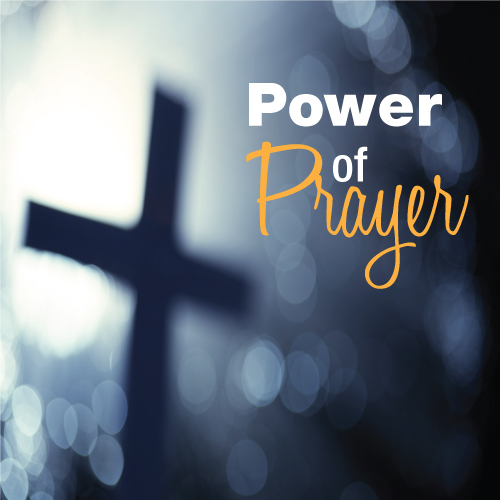St. Mark Prayer Chain
Would you like to be a part of the St. Mark prayer chain? Prayer requests come into the church office weekly. As we receive prayer requests we would send out an e-mail and ask that you pray daily for the persons mentioned in the requests. All requests are to remain confidential. Please e-mail melinda@stmarklc.com if you would like to participate in the prayer chain. “Be anxious for nothing, but in everything by prayer and supplication, with thanksgiving, let your requests be made known to God.” Colossians 4:2.

 table div table+table+table+table+table+table+table+table+table+table+table+table+table+table+table+table+table+table+table+table+table+table+table+table+table+table+table+table+table+table+table+table+table+table+table+table+table+table+table+table+table+table+table+table+table+table+table+table+table+table+table+table+table+table+table+table div table{width:100%;padding:0}table div table+table+table+table+table+table+table+table+table+table+table+table+table+table+table+table+table+table+table+table+table+table+table+table+table+table+table+table+table+table+table+table+table+table+table+table+table+table+table+table+table+table+table+table+table+table+table+table+table+table+table+table+table+table+table+table div table img{width:96.23%;padding:0;float:none}table div table+table+table+table+table+table+table+table+table+table+table+table+table+table+table+table+table+table+table+table+table+table+table+table+table+table+table+table+table+table+table+table+table+table+table+table+table+table+table+table+table+table+table+table+table+table+table+table+table+table+table+table+table+table+table+table div table td{width:100%;padding:0 1.88% 18px}/* styles */Sign up for Altar Flowers There are some dates still available to sponsor a flower arrangement on Sundays. This can be in honor or memory of someone or just because. The price for one bouquet is \$35. To sign up for flowers you can call the office at 815-398-3557, or click on this link. If you sign up online, put your memory or honor of in the comment line and pay here or write a check to St. Mark. If you have the mobile app you can do all of it on there.
You may take your flowers home after the service or stop by the office during the week to pick them up.

 table div table+table+table+table+table+table+table+table+table+table+table+table+table+table+table+table+table+table+table+table+table+table+table+table+table+table+table+table+table+table+table+table+table+table+table+table+table+table+table+table+table+table+table+table+table+table+table+table+table+table+table+table+table+table+table+table+table+table div table{width:100%;padding:0}table div table+table+table+table+table+table+table+table+table+table+table+table+table+table+table+table+table+table+table+table+table+table+table+table+table+table+table+table+table+table+table+table+table+table+table+table+table+table+table+table+table+table+table+table+table+table+table+table+table+table+table+table+table+table+table+table+table+table div table img{width:96.23%;padding:0;float:none}table div table+table+table+table+table+table+table+table+table+table+table+table+table+table+table+table+table+table+table+table+table+table+table+table+table+table+table+table+table+table+table+table+table+table+table+table+table+table+table+table+table+table+table+table+table+table+table+table+table+table+table+table+table+table+table+table+table+table div table td{width:100%;padding:0 1.88% 18px}/* styles */## Youth Ministry

 table div table+table+table+table+table+table+table+table+table+table+table+table+table+table+table+table+table+table+table+table+table+table+table+table+table+table+table+table+table+table+table+table+table+table+table+table+table+table+table+table+table+table+table+table+table+table+table+table+table+table+table+table+table+table+table+table+table+table+table+table div table{width:100%;padding:0}table div table+table+table+table+table+table+table+table+table+table+table+table+table+table+table+table+table+table+table+table+table+table+table+table+table+table+table+table+table+table+table+table+table+table+table+table+table+table+table+table+table+table+table+table+table+table+table+table+table+table+table+table+table+table+table+table+table+table+table+table div table img{width:96.23%;padding:0;float:none}table div table+table+table+table+table+table+table+table+table+table+table+table+table+table+table+table+table+table+table+table+table+table+table+table+table+table+table+table+table+table+table+table+table+table+table+table+table+table+table+table+table+table+table+table+table+table+table+table+table+table+table+table+table+table+table+table+table+table+table+table div table td{width:100%;padding:0 1.88% 18px}/* styles */High School Youth Group “kicks” off this
Sunday, September 12th. 6:30-8:00 P.M.
Questions? Melinda Alekna 815-871-0390 or e-mail melinda@stmarklc.com

 table div table+table+table+table+table+table+table+table+table+table+table+table+table+table+table+table+table+table+table+table+table+table+table+table+table+table+table+table+table+table+table+table+table+table+table+table+table+table+table+table+table+table+table+table+table+table+table+table+table+table+table+table+table+table+table+table+table+table+table+table+table+table div table{width:100%;padding:0}table div table+table+table+table+table+table+table+table+table+table+table+table+table+table+table+table+table+table+table+table+table+table+table+table+table+table+table+table+table+table+table+table+table+table+table+table+table+table+table+table+table+table+table+table+table+table+table+table+table+table+table+table+table+table+table+table+table+table+table+table+table+table div table img{width:96.23%;padding:0;float:none}table div table+table+table+table+table+table+table+table+table+table+table+table+table+table+table+table+table+table+table+table+table+table+table+table+table+table+table+table+table+table+table+table+table+table+table+table+table+table+table+table+table+table+table+table+table+table+table+table+table+table+table+table+table+table+table+table+table+table+table+table+table+table div table td{width:100%;padding:0 1.88% 18px}/* styles */## Children's MinistrySunday School Begins September 19
Welcome back to Sunday school – in person! Hooray!

Sunday school will meet weekly from 10:10-10:50 a.m. Families are encouraged to worship together at either the 9:00 or 11:00 service.

Following guidance for children’s settings here in Illinois, all children and adults are required to wear masks in the Sunday school space regardless of vaccination status. As the situation changes, we will adjust as well.

Register here and get excited for Sunday school! And as always, contact julie@stmarklc.com for more details.

 table div table+table+table+table+table+table+table+table+table+table+table+table+table+table+table+table+table+table+table+table+table+table+table+table+table+table+table+table+table+table+table+table+table+table+table+table+table+table+table+table+table+table+table+table+table+table+table+table+table+table+table+table+table+table+table+table+table+table+table+table+table+table+table+table+table div table{width:100%;padding:0}table div table+table+table+table+table+table+table+table+table+table+table+table+table+table+table+table+table+table+table+table+table+table+table+table+table+table+table+table+table+table+table+table+table+table+table+table+table+table+table+table+table+table+table+table+table+table+table+table+table+table+table+table+table+table+table+table+table+table+table+table+table+table+table+table+table div table img{width:96.23%;padding:0;float:none}table div table+table+table+table+table+table+table+table+table+table+table+table+table+table+table+table+table+table+table+table+table+table+table+table+table+table+table+table+table+table+table+table+table+table+table+table+table+table+table+table+table+table+table+table+table+table+table+table+table+table+table+table+table+table+table+table+table+table+table+table+table+table+table+table+table div table td{width:100%;padding:0 1.88% 18px}/* styles */## Our St. Mark members:

Don Wasson, John Lund, Dennis Clark, Linda Vivian, Jack Sowl, Lorine Kortus, Jan Cotter, Roger Lind, Diane Swanson, Sherri Lindquist, Bette Patterson, Keith Haggestad.

## Family and friends of St. Mark members:

Clyde Webster, Bob Pollock, Ezra Mark Wiegel, Ed Benfield, Conrad Semmelroth, Nici King, Vicki Kalnins, Debbie Adams, Wanda Adamson, Milane Bagel, Janet Webster, Rae Ann McIntire, Linda Johnson, Eva Love Sherbondy, Norma Comstock, Carolyn King, Ashton Henley.

## Those serving in the military and their families:

Hunter Haggestad, Tristan Davenport, Scot Hornick,
Bailey Panjkovich, Jim Tammen, Brandon Ballenger, Taylor Eversole, Dean Barron.

Strengthen and encourage those in public health services and in the medical profession: care-givers, nurses, attendants, doctors, all who commit themselves to care for the sick and their families.

 table div table+table+table+table+table+table+table+table+table+table+table+table+table+table+table+table+table+table+table+table+table+table+table+table+table+table+table+table+table+table+table+table+table+table+table+table+table+table+table+table+table+table+table+table+table+table+table+table+table+table+table+table+table+table+table+table+table+table+table+table+table+table+table+table+table+table+table+table div table{width:100%;padding:0}table div table+table+table+table+table+table+table+table+table+table+table+table+table+table+table+table+table+table+table+table+table+table+table+table+table+table+table+table+table+table+table+table+table+table+table+table+table+table+table+table+table+table+table+table+table+table+table+table+table+table+table+table+table+table+table+table+table+table+table+table+table+table+table+table+table+table+table+table div table img{width:96.23%;padding:0;float:none}table div table+table+table+table+table+table+table+table+table+table+table+table+table+table+table+table+table+table+table+table+table+table+table+table+table+table+table+table+table+table+table+table+table+table+table+table+table+table+table+table+table+table+table+table+table+table+table+table+table+table+table+table+table+table+table+table+table+table+table+table+table+table+table+table+table+table+table+table div table td{width:100%;padding:0 1.88% 18px}/* styles *//* styles */ Office Hours Monday through Friday 9:00 A.M. - 4:00 P.M. Telephone: 815-398-3557 Publications St. Mark Memos, the weekly e-newsletter, is distributed each Thursday. The deadline for submission is Monday by 3:00 P.M. Please click to send submissions. Worship Services Miss a Sunday at St. Mark? Please visit our website and watch the service online. Please click to watch.
 table div table+table+table+table+table+table+table+table+table+table+table+table+table+table+table+table+table+table+table+table+table+table+table+table+table+table+table+table+table+table+table+table+table+table+table+table+table+table+table+table+table+table+table+table+table+table+table+table+table+table+table+table+table+table+table+table+table+table+table+table+table+table+table+table+table+table+table+table+table+table div table{width:100%;padding:0}table div table+table+table+table+table+table+table+table+table+table+table+table+table+table+table+table+table+table+table+table+table+table+table+table+table+table+table+table+table+table+table+table+table+table+table+table+table+table+table+table+table+table+table+table+table+table+table+table+table+table+table+table+table+table+table+table+table+table+table+table+table+table+table+table+table+table+table+table+table+table div table img{width:96.23%;padding:0;float:none}table div table+table+table+table+table+table+table+table+table+table+table+table+table+table+table+table+table+table+table+table+table+table+table+table+table+table+table+table+table+table+table+table+table+table+table+table+table+table+table+table+table+table+table+table+table+table+table+table+table+table+table+table+table+table+table+table+table+table+table+table+table+table+table+table+table+table+table+table+table+table div table td{width:100%;padding:0 1.88% 18px}/* styles */